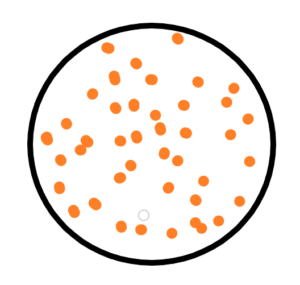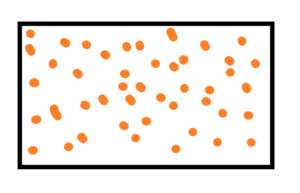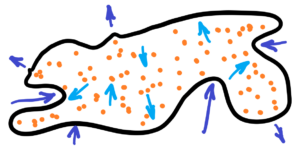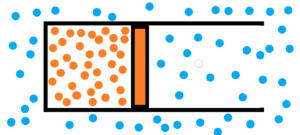### Thermodynamics  – mechanics of chaotic behaviour of particles

The basis of thermodynamics and indeed of the whole world is chaos. Chaos in a closed space. Chaos of moving particles, molecules, atoms. This chaos can be converted into mechanical work by the controlled expansion of initially chaotically moving particles. See an engine piston or a turbine blade.Let’s have a model – a closed box with N particles. Initial conditions – all particles have the same velocity. After some time we find that the velocities of the particles are different from each other. The speed of movement of particles in a closed space varies – from almost zero speed to maximum speed. There is a kind of average particle velocity that is close to the value at the initial conditions.

the velocity distribution of particles depends on :

– the volume of the box
– the size of particles (very interesting model with particles like ideal points with zero size – these never meet each other)
– the number of particles

particle size is relative to the size of the box

The rule is – the higher the speed – the shorter the duration. The same for the lowest speed. If we want to keep the lowest velocity or high velocity of a particle for a longer time – we would have to isolate the particle from other particles. Perhaps by expanding space or otherwise. See the rubber sheet thermodynamics in two dimensional space below.

See the image below – thermodynamics of rubber sheet, resp. of elastic membraneThermodynamics of rubber sheet – elastic membrane full of chaotically moving particles. The disadvantage of isolating a particle is that we can keep its velocity for a longer time, but we cannot change it. Thus, it would be better to adequately stretch the elastic membrane according to how we want to accelerate the particle or, on the contrary, to decelerate it. Which leads to very interesting results.

Go back to thermodynamics before we begin to solve the thermodynamics of an elastic membrane or an elastic volume. How to describe purely chaotic processes? It is impossible to use mathematics. Mathematics doesn’t have a chance in the case of pure randomness. Mathematics has nothing to go on. But thermodynamics exists in clearly given mathematical equations. So the equation pV = nRT, very familiar equation for engineers. Sure, but there are differences. If we have one chaotic environment, we won’t be able to study anything, we won’t be able to describe anything, and we won’t be able to predict or verify anything. See first image below – only one chaotic environment.So we need to have at least two different chaotic environments. One environment will be the chaotic motion of air molecules in the atmosphere and the other environment will be the chaotic motion of molecules in a closed cylinder with a piston. See a second upper image.
We will ignore temperature for the first time and examine what is called pressure and volume. It is possible to think like this and mainly to measure – volume and pressure – the weight of the mass relative to the given piston area. We obtain the equation p1V1 = p2V2. The multiplication of pressure and volume before expansion is equal to the multiplication of pressure and volume after expansion. Let us remember that we only know ratios and not absolute values. What is the absolute value of chaotic motion – relative to what? Relative to zero? Relative to what zero? To the zero of particles? But zero is not even in a vacuum – see the exclusion principle. Not to mention – relative to zero, everything is infinity!  And each unit we have chosen as the base unit of measurement is just a chosen ratio, the ratio between the measured value and the chosen (unit) value. Very important remark for next consideration in thermodynamics – expecially absolute temeperature, absolute pressure, absolute volume, absolute energy, absolute entropy, absolute enthalpy, etc. But the most important remark is about at least two chaotic environments with different characteristics to each other. For example, the expansion of the piston inside the steam cylinder, which is surrounded by dense, viscous oil , such expansion is different to the expansion of a piston inside a steam cylinder in an ambient atmosphere. Not to mention the change in the viscosity especially in the case of a non-Newtonian fluid.

See a cylinder with moving piston inside it. There are two different chaotical environments. See image below.1) atmosphere filled with chaotically moving gas molecules – see blue dots
2) chaotically moving gas particles enclosed by a piston in the cylinder volume – see orange dots

Both the gas and the surrounding atmosphere are purely chaotic environments. In both environments, molecules collide. But in the case of a gas, we see that the collisions among gas molecules are more violent and more frequent compared to the molecules of the surrounding atmosphere. A gas enclosed by a piston in a cylinder will have tendency to expand relative to the atmosphere until the violence and frequency of collisions among the molecules are equal. Not to mention the final damping oscillations. See image below.How to measure how to evaluate the violence and frequency of molecular collisions?
Some base units need to be chosen. See next image below.To put a unit weight on the piston. How many units times the frequency and violence of collisions. At least for the beginning.

to be continued next time …

Steam engines, turbines, pumps, motors, details as valves, propellers etc.